1. Definition; Equations

Latitude is the north-south component of a line; departure the east-west. North latitudes are positive, South are negative; similarly East departures are positive, West are negative.(a) The latitude of line AB is North (+), its departure is East (+). (b) The latitude of line CD is South (-), its departure is West (-). Figure D-1 Latitudes and Departures

Latitude (Lat) and Departure (Dep) are computed from: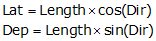Equation D-1

Dir can be either a bearing angle, Figure D-2(a), or azimuth angle, Figure D-2(b).(a) LatAB is North DepAB is East (b) LatBA is South DepBA is West Figure D-2 Bearings or Azimuths

Because a bearing angle never exceeds 90°, the Lat and Dep equations will always return positive values.Sin(0°) to Sin(90°) ranges from 0 to +1.0 Cos(0°) to Cos(90°) ranges from +1.0 to 0 The correct mathematical sign for the Lat and Dep come from the bearing quadrant. A bearing of S 47°35' E has a negative Lat (South) and a positive Dep (East). Figure D-3 Quadrants

An azimuth angle ranges from 0° to 360°, so the sine and cosine return the correct signs on the Lat and Dep.

ExamplesFigure D-4 NE Azimuth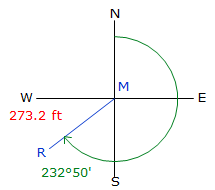Figure D-5 SW Azimuth

Reversing a line direction results in the same magnitude Lat and Dep but reversed signs:

 Line A to B Line B to A(a) (b) Figure D-6 Reverse Latitude and Departure

2. Closure

On a closed loop traverse, the sum of the Lats should equal zero as should the sum of the Deps.

Closure condition:Equation D-2Figure D-7
Perfect Closed Loop

Most traverses won’t close perfectly due to the presence of errors. If the field crew followed careful procedures and compensated distance measurements for atmospheric conditions, only random errors prevent achieving perfect closure:event achieving perfect closure:

Actual closure:Equation D-3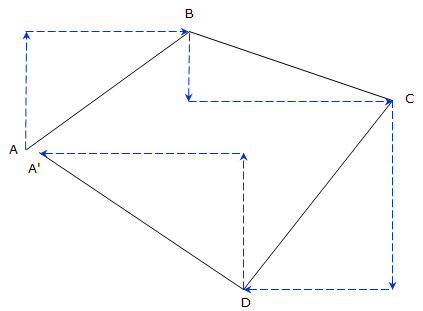Figure D-8
Cumulative Random ErrorsFigure D-9 Linear Closure

The Linear Closure, LC, is the total misclosure distance. It is computed from the Lat and Dep errors:Equartion D-4

Traverse precision is a function of the linear closure and the total survey distance. The precision is stated as a relative value, usually as 1 unit of error per D units measured:Equation D-5

What is allowable minimum precision? It depends on the survey purpose. Traditional first order surveys have a required precision of 1/100,000; in Wisconsin a property survey must be at least 1/3000. In order to achieve a formal minimum precision, specific equipment and field procedures must be used which match that precision requirement. Achieving a minimum precision should be by design not by accident.

3. Examples

In the following examples shown, all calculations are shown with an additional significant figure. Because these are generally intermediate computations, carrying an additional digit minimizes roundoff error in subsequent calculations.

When reporting results of an intermediate calculation, those should be stated to the correct number of significant figures so as not to imply an accuracy beyond that of the measurements.

a. Traverse with bearingsEquation D-6

Lat and Dep will always compute as positive; must assign correct mathematical sign based on the bearing quadrant.

Figure D-10
Bearing Traverse

Line AB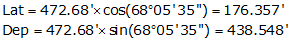Because the bearing is South and West, the Lat and Dep are -176.357' and -438.548' respectively.

Line BCBecause the bearing is North and West, the Lat and Dep are +203.395' and -73.093' respectively.

Line CDBecause the bearing is North and East, the Lat and Dep are +192.357' and +198.651' respectively.

Line DABecause the bearing is South and East , the Lat and Dep are -219.312' and +313.065' respectively

In tabular form:

 Line Bearing Length (ft) Lat (ft) Dep (ft) AB S 68°05'35"W 472.68 -176.357 -438.548 BC N 19°46'00"W 216.13 +203.395 -73.093 CD N 45°55'20"E 276.52 +192.357 +198.651 DA S 54°59'15"E 382.24 -219.312 +313.065 sums: 1347.57 +0.083 +0.075 Distance Lat err Dep err too far N too far Eb. Traverse with azimuths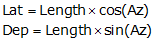Equation D-7

Lat and Dep will always compute directly with the correct sign when using azimuths.

Figure D-11
Azimuth Traverse

Line STLine TULine UVLine VSLine Azimuth Length (ft) Lat (ft) Dep (ft) ST 309°05'38" 347.00 +218.816 -269.311 TU 258°34'22" 364.55 -72.226 -357.324 UV 128°04'44" 472.74 -291.560 +372.123 VS 60°21'26" 292.94 +144.885 +254.602 sums: 1477.23 -0.085 +0.090 Distance Lat err Dep err too far S too far E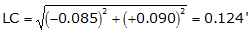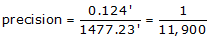c. Crossing Traverse

A four-sided parcel has two obstructed lines.Figure D-12 Parcel Boundaries

In order to create a closed traverse, the survey crew measures a crossing traverse which connects all four points.As long as a traverse closes back on its beginning point, the closer condition is still:regardless of how many times it may cross itself.   Given this traverse data, determine its closure and precision. Figure D-13 Closed Crossing Traverse

Rather than write out each Lat and Dep computation separately, we can simply set up the table and record the computations in it.

 Line Azimuth Length (ft) Lat (ft) Dep (ft) EF 133°02'45" 455.03 -310.780 +332.737 FG 24°33'35" 228.35 +207.691 +94.912 GH 241°05'15" 422.78 -204.403 -370.084 HE 349°25'20" 213.85 +307.534 -57.430 sums: 1419.28 +0.042 +0.135 Distance Lat err Dep err too far N Too far EHits: 31863